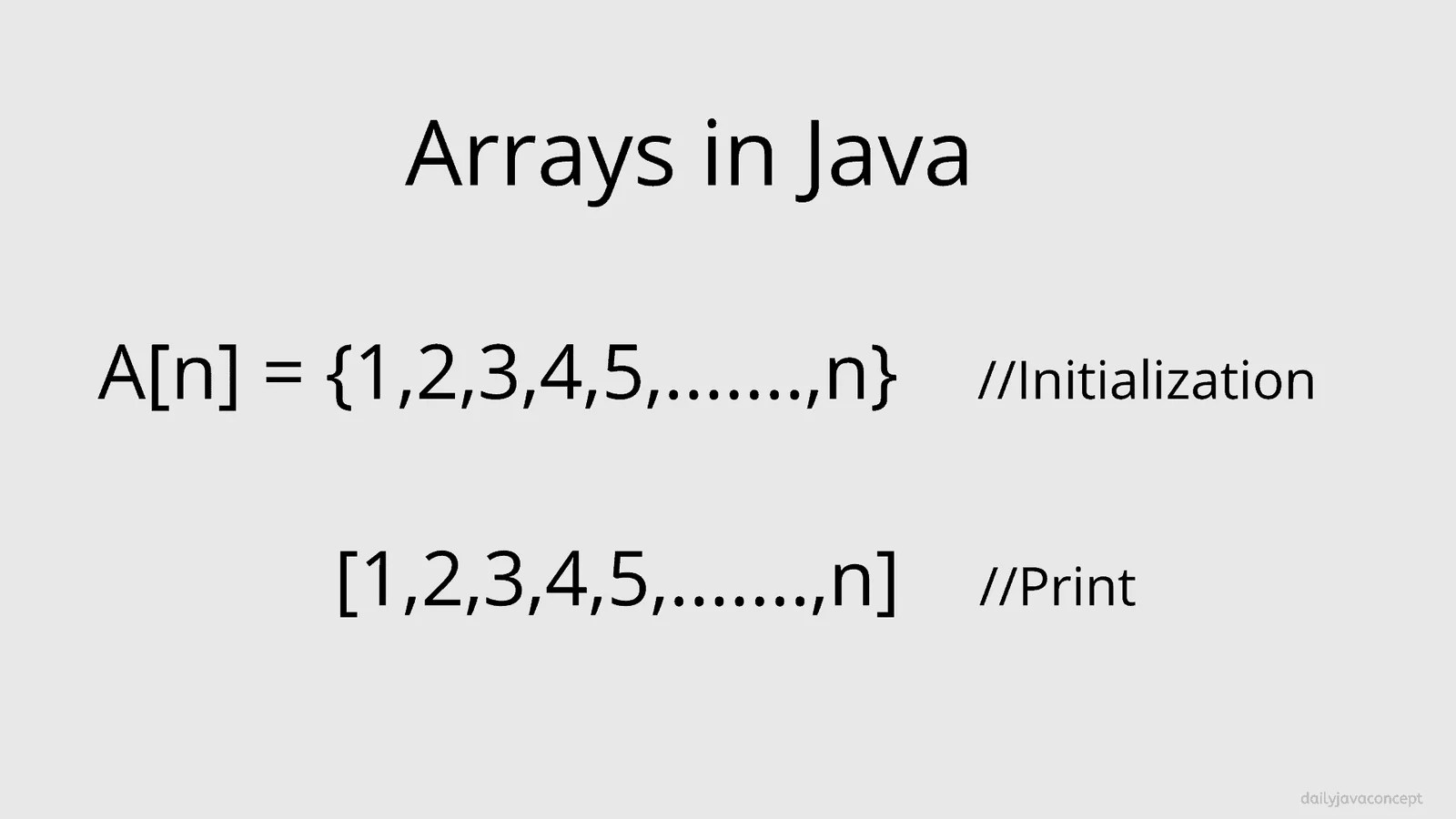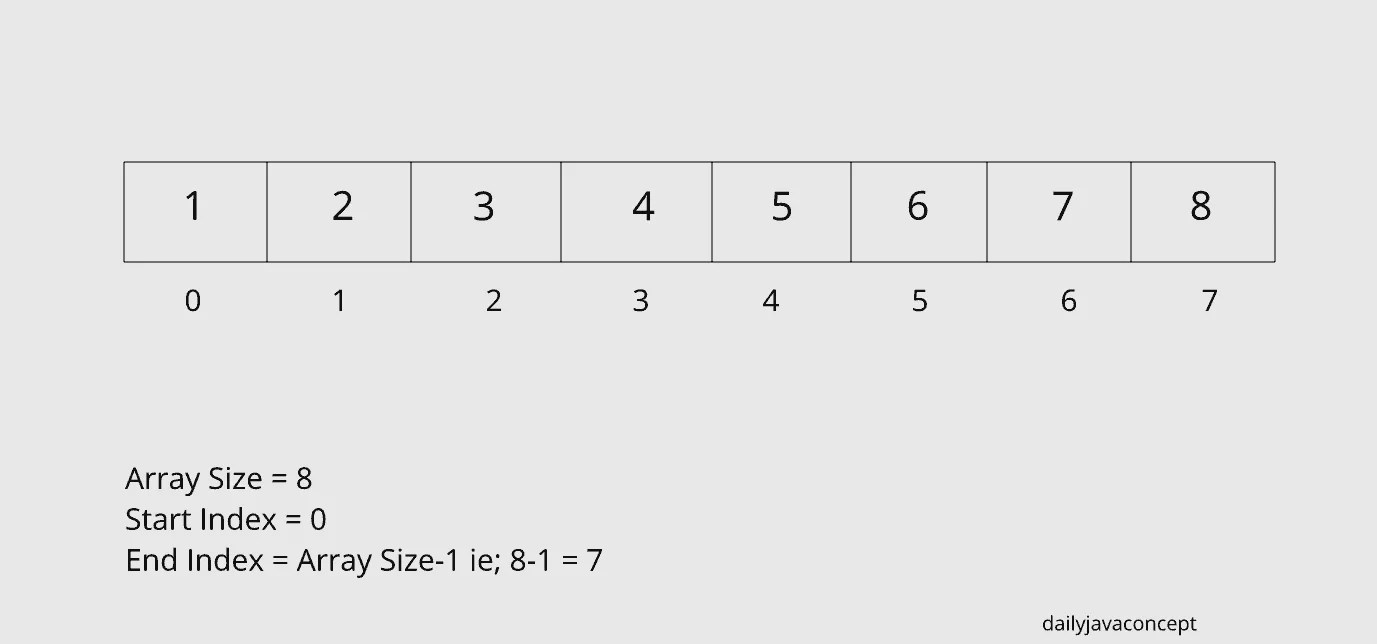# Arrays in Java

0
83### Arrays in Java

An array is a type of data structure where we can store the elements of the same type. An array can be set of Integer values, double values and string values etc. In Array, data stored in the form of the index starts with 0 and ends with n-1 where n is the size of the array.

Arrays are useful where we are going to create more similar type variable like if we are going to create 10 new int variables it takes time to create variable but in place of creating 10 new variables, we can create a new array variable that can store 10 int values.Here are some points to keep in mind about Arrays in Java:

1. An array is a Data Structure where we can store a similar kind of things.
2. An array is in the form of the index where the index starts with 0 and ends with (Array Size)-1.
3. Array size must be an integer value, not a float or other value.
4. In Java, Array can dynamically allocate memory using the new operator.
5. Array allocated with new operator initialized with their default value like  0(for Integer), false(for Boolean), null(for String and another reference type) etc.
6. An array is fixed in size, once array declared we can not increase or decrease the size of an array, so there is memory wastage in an array if we are not consuming the whole size of an array.
7. In Java, Array implements Random Access Interface that is the subclass of java.util.
8. In Java, Array can store primitive type values as well as Class Objects.
9. Primitive type values stored in Contiguous Memory locations and the class objects stored in Heap Memory.
10. In Java, Arrays can implement java.lang.Cloneable and java.io.Serializable that helps array to send over the network.

##### Creating, Initializing and Accessing Array:

Using an array in Java is a two-step process. First, you should declare an array variable with the desired type and Second, you should allocate the memory that should hold the array and then assign allocated memory to an array variable.

An array can be of two types:

• 1-Dimensional Array
• Multi-Dimensional Array
###### 1-D Array:

A 1-D array is also called a Linear array where the elements stored in linearly. first, we declare the array variable with the desired type.

```//Syntax of creating desired array variable

Data_type[] Variable_name;

or

Data_type Variable_name[];```

there is some example of creating an array variable:

```//these are the array declaration of primitive data types

int[] array_of_integers;
double[] array_of_doubles;
char[] array_of_characters;

float array_of_floats[];

//these are the array declaration of Objects
//in Java String is a class, not a data type

String[] array_of_strings;
Student array_of_students[];```

After declaring the array we must allocate the memory for array variables and then that allocated memory assigns to their respective array variable.

In Java, an array can be initialized dynamically using the new operator.

```//Syntax of allocating memory to an array variable

array_variable = new Data_types[size_of_array];

or

array_variable = new array_variable[size_of_array];```

There is some example of allocating memory to their respective array variable:

```array_of_integers = new int;

array_of_doubles = new double;

array_of_characters = new char;

//same for object types

array_of_strings = new String;

array_of_students = new array_of_students;```

You can also define array directly like:

`int[] array_of_integers = {1,2,3,4,5,6,7,8,9,10};`

but it’s not very good practice for real-world problems.

After creating the array and also initializing the array access the array using for loop. we can also use other loops like a for-each loop or while loop but for loop is more convenient for accessing array elements.

Here, we are writing a Java program that describes you about creating an array, allocating memory and accessing the array elements.

```import java.util.Scanner;

public class Describing_Array {
public static void main(String[] args) {

int[] array_of_integers; //declaring array variable

Scanner sc = new Scanner(System.in);
//first we ask the size of array to user

System.out.println("Enter the size of array : ");
int size = sc.nextInt();

array_of_integers = new int[size]; //dynamically allocating the memory to array variable

// next steps is to accessing array
//store the values in array

System.out.println("Enter " + size + " elements : ");

for (int i = 0; i < size; i++) {
array_of_integers[i] = sc.nextInt();
}

//Print the array elements

System.out.println("Array elements are : ");

for (int i = 0; i < array_of_integers.length; i++) {

System.out.println(array_of_integers[i]);

}

//we can directly print the array elements using the index
System.out.println("Print array element at the index 1 : ");
System.out.println(array_of_integers);

/*if array does not have that index then it throws ArrayIndexOutOfBoundsException*/
//System.out.println("Showing ArrayIndexOutOfBoundsException : ");
/*In array there are only 5 elements 0 to 4, now we are accessing the 5th elements that throws the Exception*/
// this line throw the Exception if you want to see, just remove the comment and see the Exception
//System.out.println(array_of_integers);

//Increasing the array elements by 1
for (int i = 0; i < array_of_integers.length; i++) {

array_of_integers[i] = array_of_integers[i] + 1;

}

// now showing the updated array
System.out.println("Updated Array : ");
for (int i = 0; i < array_of_integers.length; i++) {

System.out.println(array_of_integers[i]);

}

}
}```

###### Multidimensional Array:Here we are showing how to declare and initialize the multidimensional array :

```//Syntax for declaring and allocating the

int[][] multi_d_array_integer = new int[row][column];```

here, we are writing a java program to describe how to access the multidimensional array in java:

```public class Describing_Array {

public static void main(String[] args) {

//declaring array variable and initializing the array
int[][] array_of_integers = {{1, 2, 3}, {4, 5, 6}, {7, 8, 9}};

//Printing the array elements

for (int i = 0; i < array_of_integers.length; i++) {
for (int j = 0; j < array_of_integers[i].length; j++) {

System.out.println("Array[" + i + "][" + j + "] = " + array_of_integers[i][j]);
}
}

}
}```

I hope you find this post useful. If you find any anything incorrect in this post or you have any doubts regarding this post. Please let us know in the comment section.

You may also learn these to build the Knowledge: